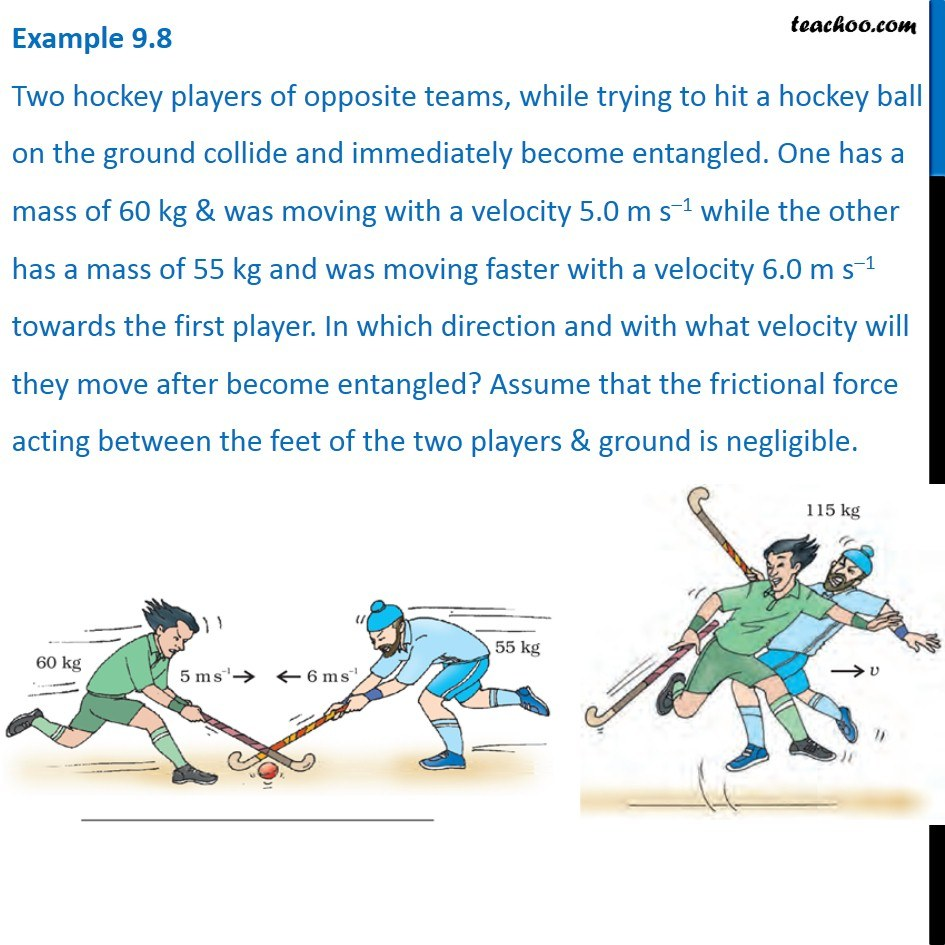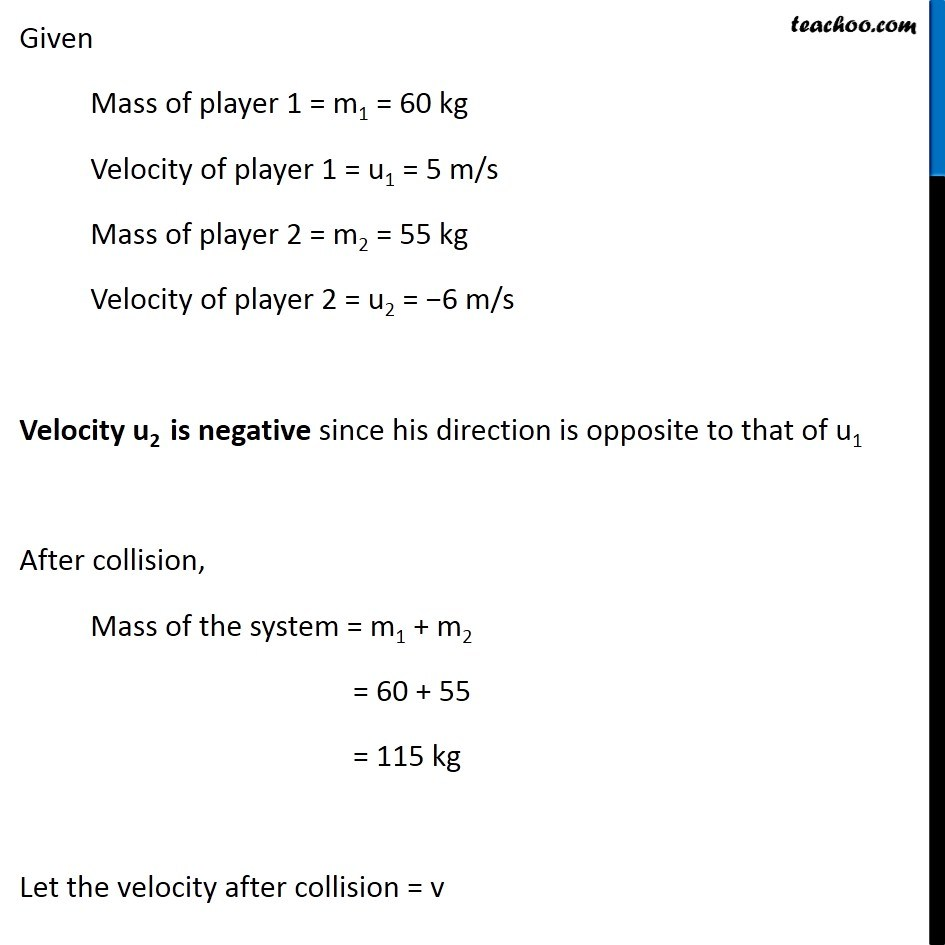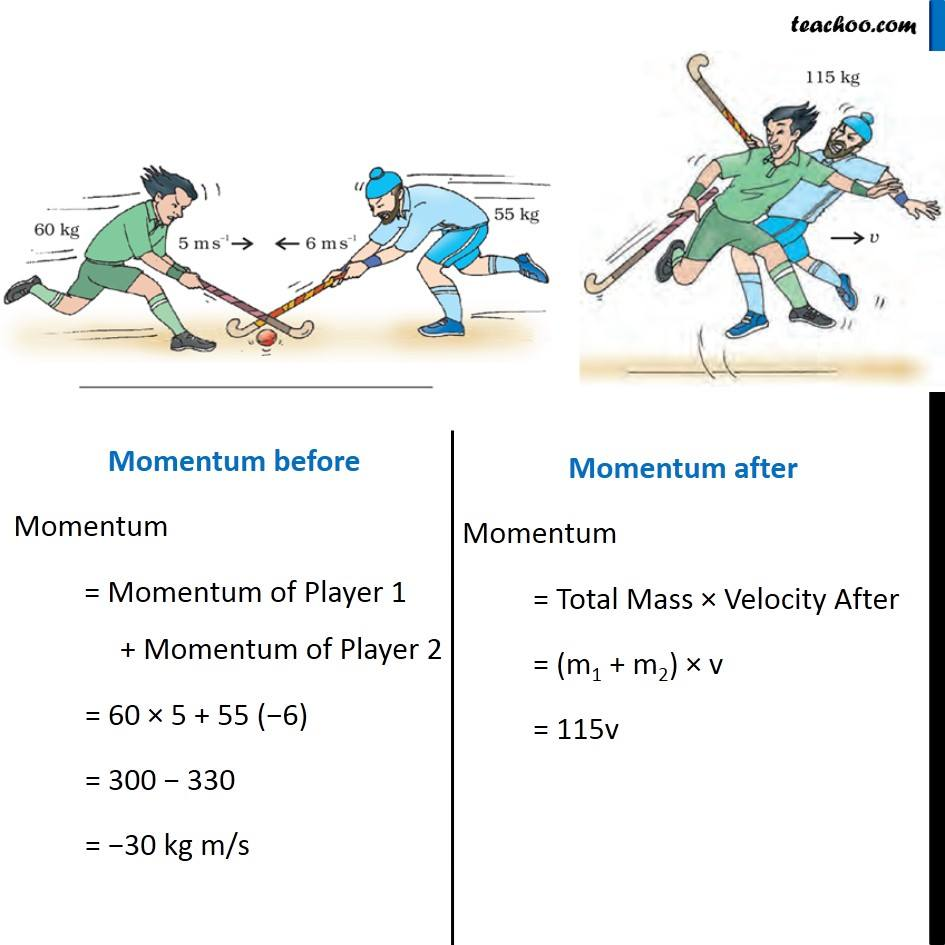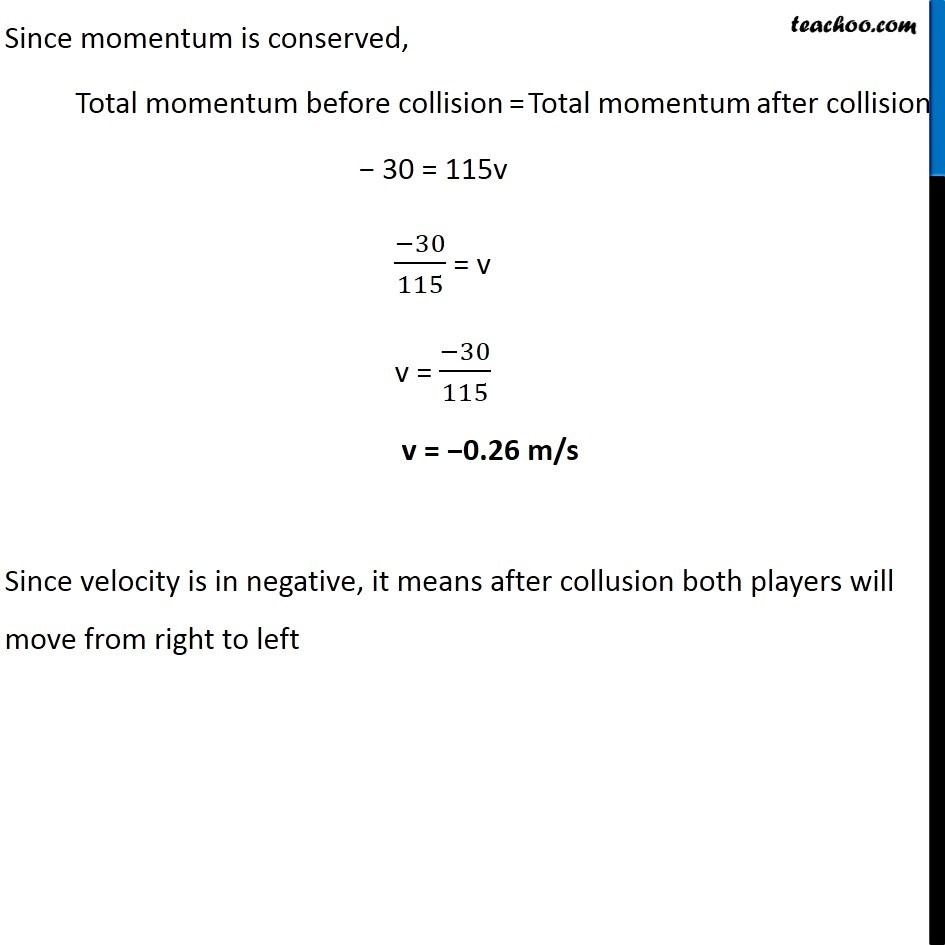Examples from NCERT Book

Class 9
Chapter 9 Class 9 - Force and Laws Of Motion### Transcript

Example 9.8 Two hockey players of opposite teams, while trying to hit a hockey ball on the ground collide and immediately become entangled. One has a mass of 60 kg & was moving with a velocity 5.0 m s–1 while the other has a mass of 55 kg and was moving faster with a velocity 6.0 m s–1 towards the first player. In which direction and with what velocity will they move after become entangled? Assume that the frictional force acting between the feet of the two players & ground is negligible. Given Mass of player 1 = m1 = 60 kg Velocity of player 1 = u1 = 5 m/s Mass of player 2 = m2 = 55 kg Velocity of player 2 = u2 = −6 m/s Velocity u2 is negative since his direction is opposite to that of u1 After collision, Mass of the system = m1 + m2 = 60 + 55 = 115 kg Let the velocity after collision = v Momentum before Momentum = Momentum of Player 1 + Momentum of Player 2 = 60 × 5 + 55 (−6) = 300 − 330 = −30 kg m/s Momentum after Momentum = Total Mass × Velocity After = (m1 + m2) × v = 115v Since momentum is conserved, Total momentum before collision = Total momentum after collision − 30 = 115v (−30)/115 = v v = (−30)/115 v = −0.26 m/s Since velocity is in negative, it means after collusion both players will move from right to left